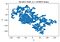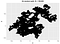# Random Walk in Two-Dimension

## The random walk is an important model for stochastic processes with applications in physics, statistics, finance, etc.

The random walk is an example of a stochastic or random process. Here, the outcome at time t+1 is independent of the outcome at time t. Random walks have applications in several disciplines such as mathematics, physics, biology, chemistry, finance, etc. For example, a random walk can be used in finance to model stock prices, or in physics to model Brownian motion.

In this article, we present an example of a discrete random walk in two-dimension.

Problem definition: Suppose we start at the origin on a two-dimensional (2D) lattice on the x-y plane, what is the location of the random walk after n steps?

`Start at the origin (x = 0, y = 0)Toss a hypothetical die with 4 faces (1, 2, 3, 4)If the die returns 1, take a right stepIf the die returns 2, take a left stepIf the die returns 3, take a step upIf the die returns 4, take a step downRepeat the process for all n stepsGenerate a visualization to show trajectory of random walk`
`import numpyimport pylabimport randomn = 100000x = numpy.zeros(n)y = numpy.zeros(n)for i in range(1, n):    val = random.randint(1, 4)    if val == 1:        x[i] = x[i - 1] + 1        y[i] = y[i - 1]    elif val == 2:        x[i] = x[i - 1] - 1        y[i] = y[i - 1]    elif val == 3:        x[i] = x[i - 1]        y[i] = y[i - 1] + 1    else:        x[i] = x[i - 1]        y[i] = y[i - 1] - 1`
`pylab.title("Random Walk (\$n = " + str(n) + "\$ steps)")pylab.plot(x, y)pylab.xlabel("x", size=12)pylab.ylabel("y", size=12)pylab.savefig("rand_walk"+str(n)+".png",bbox_inches="tight",dpi=600)pylab.show()`Figure 1. Output 1 of discrete random walk in 2D with 100,000 steps.Figure 2. Output 2 of discrete random walk in 2D with 100,000 steps.

We observe that the trajectory is random and different each time we run the code.

In summary, we’ve introduced a very simple example of a discrete random walk in 2D. Random walks are very important in probability theory and have applications in mathematics, physics, biology, chemistry, finance, etc.

## Personal Finance Analytics

Providing tips to help people learn about money, avoid debt, and invest using data-driven strategies

Written by

## Benjamin Obi Tayo Ph.D.

Physicist, Data Science Educator, Writer. Interests: Data Science, Machine Learning, AI, Python & R, Personal Finance Analytics, Materials Sciences, Biophysics

## Personal Finance Analytics

Providing useful tips to help individuals and families to be smart about money, avoid debt, and invest using data-driven strategies

Written by

## Benjamin Obi Tayo Ph.D.

Physicist, Data Science Educator, Writer. Interests: Data Science, Machine Learning, AI, Python & R, Personal Finance Analytics, Materials Sciences, Biophysics## Personal Finance Analytics

Providing useful tips to help individuals and families to be smart about money, avoid debt, and invest using data-driven strategies

## More From Medium

Medium is an open platform where 170 million readers come to find insightful and dynamic thinking. Here, expert and undiscovered voices alike dive into the heart of any topic and bring new ideas to the surface. Learn more

Follow the writers, publications, and topics that matter to you, and you’ll see them on your homepage and in your inbox. Explore

If you have a story to tell, knowledge to share, or a perspective to offer — welcome home. It’s easy and free to post your thinking on any topic. Write on Medium